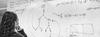# 1st Semester 2008/09: Rudiments of Category Theory

Instructors
Raul Leal

http://staff.science.uva.nl/~rlealrod/categoriesproject.html
ECTS
6
Description
Goal. This aim of this project is to introduce students to category theory and to categorical reasoning. This project is a preparation for more advanced study of the field or/and its applications to logic. After the project the students will be able to study (advanced) topics with more classical books like McLane or Adamek et al.
Category theory and set theory are foundational frameworks for mathematics. Each of these frameworks has a different approach to mathematical objects; the former uses a synthetic approach, the latter uses an analytical approach.

In this project we will formalize nae mathematics with the language of category theory. It is worth the effort to study this idea because it provides a unified guide to approaching constructions and problems in the science of space and quantity. We will focus on the notions of diagram, functor, natural transformation, and adjunction; the latter being one of the most powerful tools Category Theory has introduced to mathematics.

Assessment
Students can choose to be evaluated either on the base of basic and advanced exercises or on the base of basic exercises and a final paper. The former option is assumed by default.
References
• Category Theory Lecture Notes by D. Turi.
• Sets for Mathematics by F. W. Lawvere & R. Rosebrugh.
• Conceptual Mathematics: A First Introduction to Categories by F. W. Lawvere & S. H. Schanuel.
• Topoi: The categorical analysis of Logic by R. Goldblatt.